# Freehand segmentation in the a*-b* plane

url = 'https://blogs.mathworks.com/images/steve/2010/mms.jpg';
imshow(rgb)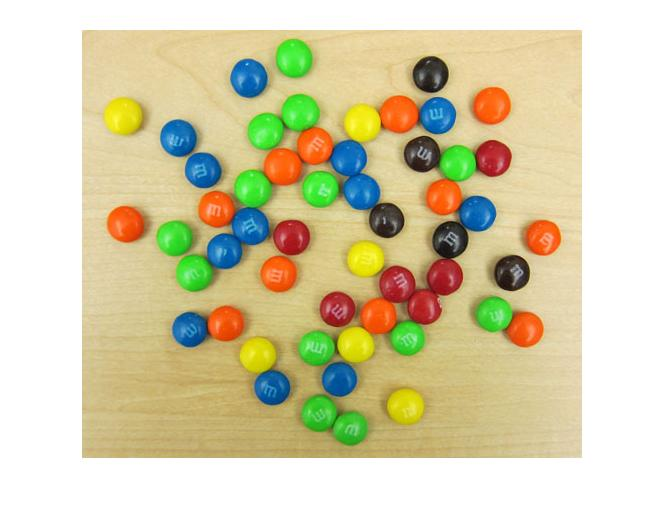Previously I showed how to convert pixel colors to the L*a*b* space and how to compute a two-dimensional histogram of the a* and b* color components: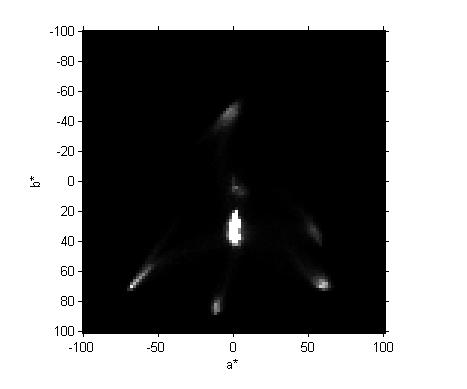Now I want to segment the image based on one of the blobs in this histogram. I used imfreehand to draw around the blob on the left-left. I can't directly show this interactive operation here, but here's a screen shot: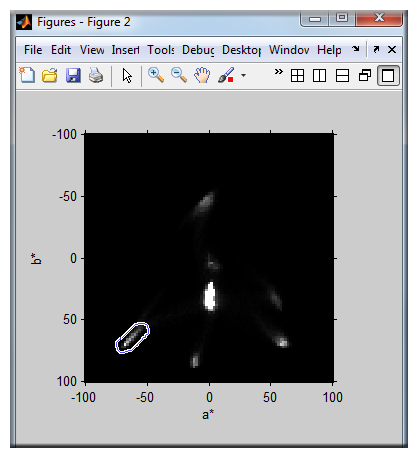Here's the code I used to draw the shape and to get the coordinates of its outline:

  h = imfreehand(gca);
% draw shape using mouse
ab = getPosition(h);

I stored the P-by-2 matrix of a*-b* coordinates in a MAT-file so I can reproduce the results in this blog post. Here's the code copy this MAT-file from its online location to a temporary location and then load it into MATLAB.

matfile_url = 'https://blogs.mathworks.com/images/steve/2011/freehand_segment.mat';
temp_matfile = [tempname '.mat'];
urlwrite(matfile_url, temp_matfile);
delete(temp_matfile)

Here are the first few saved a*-b* values:

s.ab(1:5,:)
ans =

-71.5173   65.6792
-70.9335   65.6792
-70.9335   65.0954
-70.3497   64.5116
-69.7659   63.9277



Now I want to ask the question, "Which original image pixel values have a*-b* values that fall within this shape?" I'll answer the question using inpolygon.

lab = lab2double(applycform(rgb, makecform('srgb2lab')));
a = lab(:,:,2);
b = lab(:,:,3);

in = inpolygon(a, b, s.ab(:,1), s.ab(:,2));
imshow(in)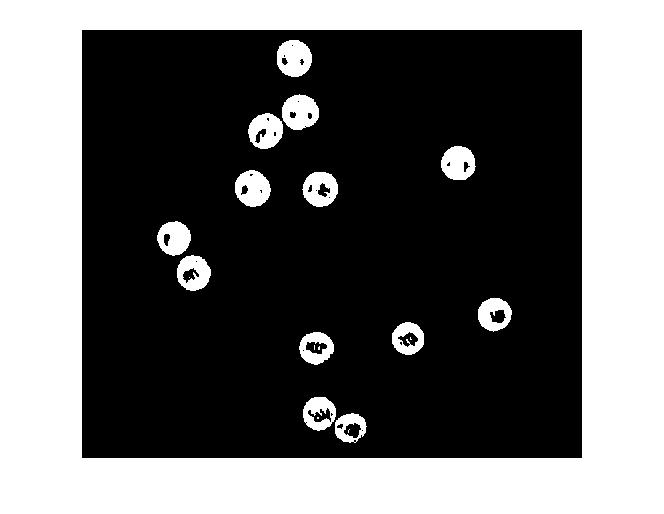That looks promising, but I'd like to visualize this result superimposed over the original image. To do that, I'll fill in the holes, find the perimeter of the result, thicken the perimeter a bit to make it easier to see, and then overlay it in black. (NOTE: imoverlay is not part of the Image Processing Toolbox. You can download it from the MATLAB Central File Exchange.)

in_filled = imfill(in, 'holes');
in_filled_perimeter = bwperim(in_filled);
in_filled_perimeter_thickened = imdilate(in_filled_perimeter, ones(3,3));

rgb2 = imoverlay(rgb, in_filled_perimeter_thickened, [0 0 0]);
imshow(rgb2)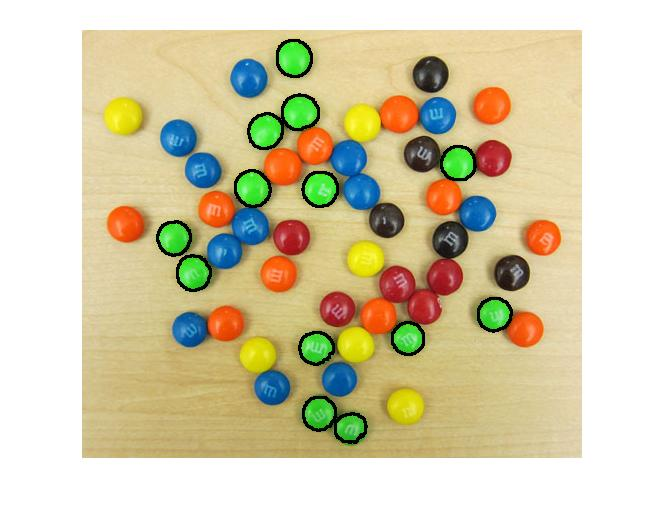Not bad! It looks like we found all the green M&Ms.

There are a couple more things I want to try with this image before giving it a rest. I think that next time I'll look at how one might implement a nearest-neighbor classifier.

Published with MATLAB® 7.11

|

### 댓글

댓글을 남기려면 링크 를 클릭하여 MathWorks 계정에 로그인하거나 계정을 새로 만드십시오.